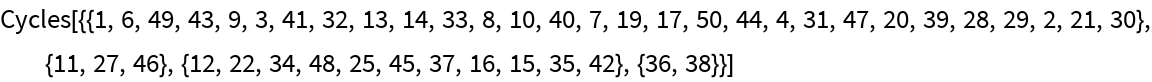#Function Repository Resource:

# DescendingSublists

Split a list at its left-to-right maxima

Contributed by: George Beck
 ResourceFunction["DescendingSublists"][list] makes sublists of list starting at its left-to-right maxima.

## Details and Options

The elements in the sublists are in the same order as the original list.
A left-to-right maximum is greater than any preceding element.
The first element of each sublist is its maximum.
The first elements of the sublists are increasing.

## Examples

### Basic Examples

Split a permutation given as a list into sublists starting at its left-to-right maximum:

 In:=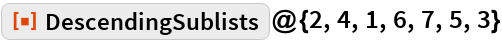Out=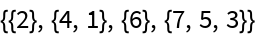The input does not have to be a permutation:

 In:=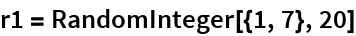Out=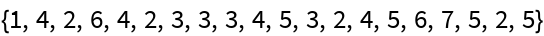In:=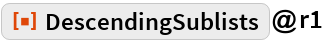Out=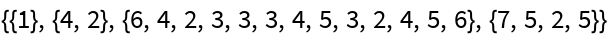Flatten to get back to the original list:

 In:=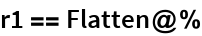Out=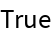### Scope

Here is a larger example:

 In:=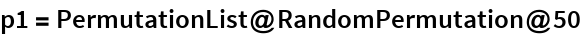Out=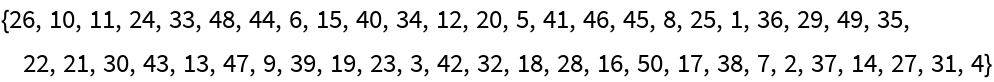In:=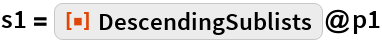Out=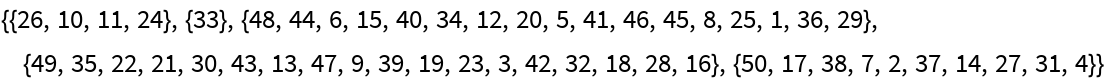Each sublist starts from its maximum:

 In:=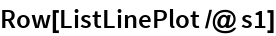Out=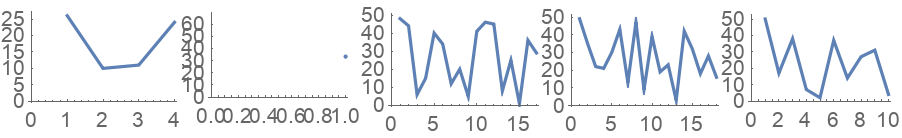The first elements are increasing:

 In:=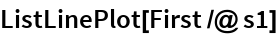Out=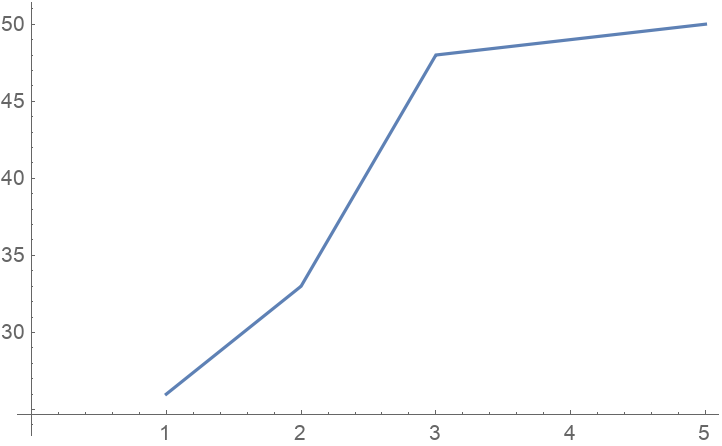The sublists of s1 can be thought of as cycle notation for the permutation, but with a different canonical form than the Wolfram Language default, where each sublist starts with its minimum:

 In:=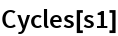Out=